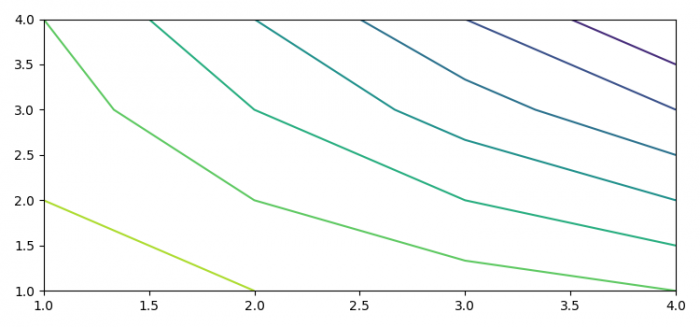# How to get coordinates from the contour in matplotlib?

To get coordinates from the contour in matplotlib, we can take the following steps −

• Set the figure size and adjust the padding between and around the subplots.
• Create lists of x, y and m with data points.
• Use plt.contour(x, y, m) to create a contour plot with x, y and m data points.
• Get the contour collections instance.
• Get the path of the collections, and print the vertices or coordinates of the contour.
• To display the figure, use show() method.

## Example

import matplotlib.pyplot as plt

plt.rcParams["figure.figsize"] = [7.50, 3.50]
plt.rcParams["figure.autolayout"] = True

x = [1, 2, 3, 4]
y = [1, 2, 3, 4]
m = [[15, 14, 13, 12], [14, 12, 10, 8], [13, 10, 7, 4], [12, 8, 4, 0]]

cs = plt.contour(x, y, m)

for item in cs.collections:
for i in item.get_paths():
v = i.vertices
x = v[:, 0]
y = v[:, 1]
print(x, y)
plt.show()

## Output

It will produce the following outputIn addition, it will print the coordinates of the contour on the terminal

[4.] [4.]
[4. 3.5] [3.5 4. ]
[4. 3.] [3. 4.]
[4.      3.33333333 3.     2.5 ] [2.5    3.     3.33333333 4.   ]
[4.      3. 2.66666667     2. ]  [2.     2.66666667   3.   4. ]
[4. 3. 2. 1.5] [1.5 2. 3. 4. ]
[4. 3. 2.     1.33333333 1. ] [1. 1.33333333 2. 3. 4. ]
[2. 1.] [1. 2.]